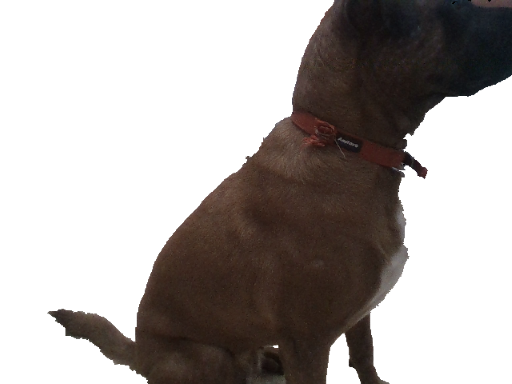# rs-grabcuts

Extracting foreground

# Overview

This example demonstrates how to enchance existing 2D algorithms with 3D data: GrabCut algorithm is commonly used for interactive, user-assisted foreground extraction.
In this demo we replace user input with initial guess based on depth data.

### 📘

How is it different from rs-align example?
rs-align is doing real-time background removal using simple masking and thresholding. This results in a fast but not a very clean results. This demo is performing pixel-level optimization to cut the foreground in the 2D image. The depth data serves only as an initial estimate of what is near and what is far.

# Example Flow

## Get Aligned Color & Depth

We start by getting a pair of spatially and temporally synchronized frames:

``````frameset data = pipe.wait_for_frames();
// Make sure the frameset is spatialy aligned
// (each pixel in depth image corresponds to the same pixel in the color image)
frameset aligned_set = align_to.process(data);
frame depth = aligned_set.get_depth_frame();
auto color_mat = frame_to_mat(aligned_set.get_color_frame());
``````

## Generate Near / Far Mask

We continue to generate pixel regions that would estimate near and far objects. We use basic morphological transformations to improve the quality of the two masks:

``````// Generate "near" mask image:
auto near = frame_to_mat(bw_depth);
cvtColor(near, near, CV_BGR2GRAY);
// Take just values within range [180-255]
// These will roughly correspond to near objects due to histogram equalization

auto far = frame_to_mat(bw_depth);
cvtColor(far, far, CV_BGR2GRAY);
// Note: 0 value does not indicate pixel near the camera, and requires special attention:
far.setTo(255, far == 0);
``````

## Invoke cv::GrabCut Algorithm

The two masks are combined into a single guess:

``````// GrabCut algorithm needs a mask with every pixel marked as either:
// BGD, FGB, PR_BGD, PR_FGB
mask.setTo(Scalar::all(GC_BGD)); // Set "background" as default guess
mask.setTo(GC_PR_BGD, far == 0); // Relax this to "probably background" for pixels outside "far" region
mask.setTo(GC_FGD, near == 255); // Set pixels within the "near" region to "foreground"
``````

We run the algorithm:

``````Mat bgModel, fgModel;
``````// Extract foreground pixels based on refined mask from the algorithm